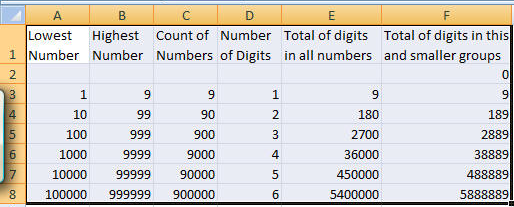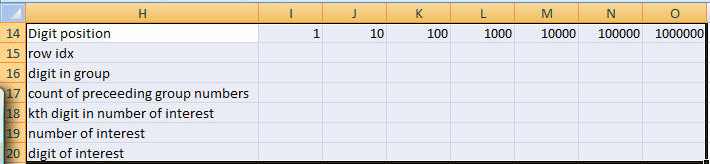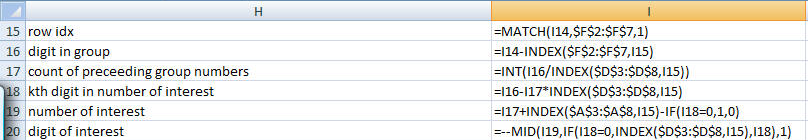﻿ Project Euler Problem 40
You are on the Home/Other Tutorials/Project Euler/Problem 40 pageShare Your

# Project Euler - Problem 40

## Problem description

An irrational decimal fraction is created by concatenating the positive integers:

0.123456789101112131415161718192021...

It can be seen that the 12thdigit of the fractional part is 1.

If dnrepresents the nthdigit of the fractional part, find the value of the following expression.

d1x d10x d100x d1000x d10000x d100000x d1000000

## Solution

The brute force VBA approach below took 200 seconds on my laptop.  Of course, I could run it on the much faster desktop in under a minute but here's how to do it by hand:

There are 9 numbers (1 through 9) with 1 digit each.  In total they account for 9 digits.

There are 90 numbers (10 through 99) with 2 digits each.  In total they account for 180 digits.  Numbers with 1 or 2 digits account for 189 digits.

There are 900 numbers (100 through 999) with 3 digits each.  In total they account for 2700 digits.  Numbers with 1, 2, or 3 digits account for 2889 digits.

The table below lists the range of numbers required to get to 1,000,000 digits.Now, in some other range, create the following:In I15:I20 enter the formulasand copy them to J:O.  The required result is the product of I20:O20.

```Option Explicit
Option Base 0

Sub Euler040()
Dim IrrNbr As String, I As Long, ProcTime As Single
I = 1
ProcTime = Timer
Do While Len(IrrNbr) < 10 ^ 6
IrrNbr = IrrNbr & CStr(I): I = I + 1
Loop
Dim MultRslt As Integer
MultRslt = 1
For I = 0 To 6
MultRslt = MultRslt * CInt(Mid(IrrNbr, 10 ^ I, 1))
Next I
Debug.Print MultRslt, Timer - ProcTime
End Sub```